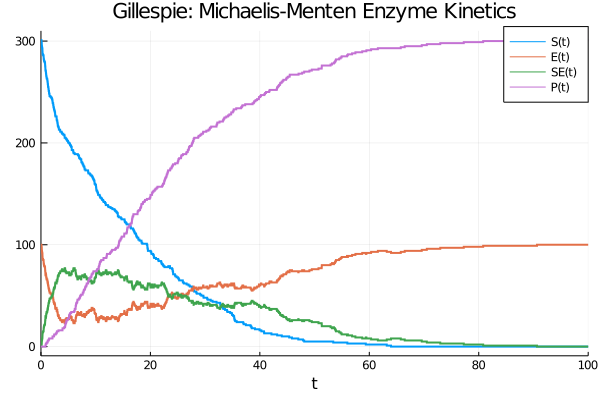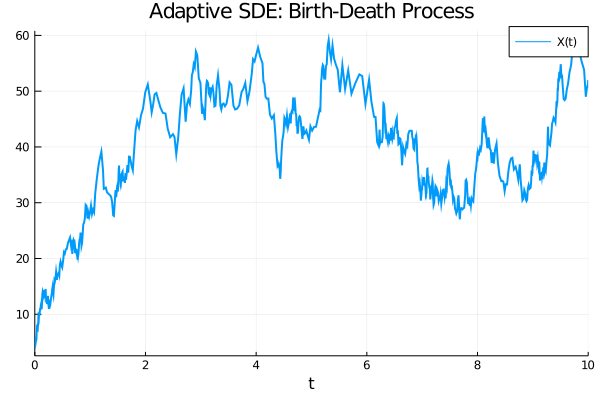## Catalyst.jl

Chemical reaction network and systems biology interface for scientific machine learning (SciML). High performance, GPU-parallelized, and O(1) solvers in open source software
Author SciML
Popularity
175 Stars
Updated Last
1 Month Ago
Started In
February 2017

# Catalyst.jl

Catalyst.jl is a domain-specific language (DSL) for high-performance simulation and modeling of chemical reaction networks. Catalyst utilizes ModelingToolkit `ReactionSystem`s, leveraging ModelingToolkit to enable large-scale simulations through auto-vectorization and parallelism. `ReactionSystem`s can be used to generate ModelingToolkit-based models, allowing easy simulation and parameter estimation of mass action ODE models, Chemical Langevin SDE models, stochastic chemical kinetics jump process models, and more. Generated models can be used with solvers throughout the broader SciML ecosystem, including higher-level SciML packages (e.g., for sensitivity analysis, parameter estimation, machine learning applications, etc.).

## New in 6.11

1. Plain text arrows "<--" and "<-->" for backward and reversible reactions are available if using Julia 1.6:

```rn = @reaction_network begin
(k1,k2), A + B <--> C
k3, 0 <-- C
end k1 k2 k3```

2. Reaction networks can be named

```rn = @reaction_network Reversible_Reaction begin
k1, A --> B
k2, B --> A
end k1 k2
nameof(rn) == :Reversible_Reaction```

Note, empty networks can no longer be created with parameters, i.e. only

```rn = @reaction_network          # uses a randomly generated name
rn = @reaction_network MyName   # is named MyName```

are allowed.

3. Compositional modeling with generated `ODESystem`s, see here for an example that composes three gene modules to make the repressilator.

## Tutorials and Documentation

For information on using the package, see the stable documentation. The in-development documentation describes unreleased features in the current master branch.

## Features

• DSL provides a simple and readable format for manually specifying chemical reactions.
• The Catalyst.jl API provides functionality for extending networks, building networks programmatically, and for composing multiple networks together.
• `ReactionSystem`s generated by the DSL can be converted to a variety of `ModelingToolkit.AbstractSystem`s, including ODE, SDE, and jump process representations.
• By leveraging ModelingToolkit, users have a variety of options for generating optimized system representations to use in solvers. These include construction of dense or sparse Jacobians, multithreading or parallelization of generated derivative functions, automatic classification of reactions into optimized jump types for Gillespie-type simulations, automatic construction of dependency graphs for jump systems, and more.
• Generated systems can be solved using any DifferentialEquations.jl ODE/SDE/jump solver, and can be used within `EnsembleProblem`s for carrying out GPU-parallelized parameter sweeps and statistical sampling. Plot recipes are available for visualizing the solutions.
• Julia `Expr`s can be obtained for all rate laws and functions determining the deterministic and stochastic terms within resulting ODE, SDE, or jump models.
• Latexify can be used to generate LaTeX expressions corresponding to generated mathematical models or the underlying set of reactions.
• Graphviz can be used to generate and visualize reaction network graphs. (Reusing the Graphviz interface created in Catlab.jl.)

## Illustrative Examples

#### Gillespie Simulations of Michaelis-Menten Enzyme Kinetics

```rs = @reaction_network begin
c1, S + E --> SE
c2, SE --> S + E
c3, SE --> P + E
end c1 c2 c3
p = (0.00166,0.0001,0.1)   # [c1,c2,c3]
tspan = (0., 100.)
u0 = [301., 100., 0., 0.]  # [S,E,SE,P]

# solve JumpProblem
dprob = DiscreteProblem(rs, u0, tspan, p)
jprob = JumpProblem(rs, dprob, Direct())
jsol = solve(jprob, SSAStepper())
plot(jsol,lw=2,title="Gillespie: Michaelis-Menten Enzyme Kinetics")```#### Adaptive SDEs for A Birth-Death Process

```using Catalyst, Plots, StochasticDiffEq, DiffEqJump
rs = @reaction_network begin
c1, X --> 2X
c2, X --> 0
c3, 0 --> X
end c1 c2 c3
p = (1.0,2.0,50.) # [c1,c2,c3]
tspan = (0.,10.)
u0 = [5.]         # [X]
sprob = SDEProblem(rs, u0, tspan, p)
ssol  = solve(sprob, LambaEM(), reltol=1e-3)
plot(ssol,lw=2,title="Adaptive SDE: Birth-Death Process")```### Required Packages

View all packages